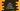# Java program to check if a number is Armstrong or not## Java program to check if a number is Armstrong or not:

In this post, we will write one Java program that will take one number as input from the user and find out if that number is an Armstrong number or not.

You will learn how to read a number from the user, what is an Armstrong number and how to check if a number is Armstrong or not.

### Definition of Armstrong number:

A positive number of length n, or total digits n is called an Armstrong number if the sum of nth powers of the digits is equal to the number itself.

For example, 153 is an Armstrong number. Because

• It has 3 digits.
• The sum of cube of each digit is 1^3 + 5^3 + 3^3 = 1 + 125 + 27 = 153, i.e. the number itself.

### Algorithm to find if a number is Armstrong number or not:

We can use the below algorithm to find if a number is Armstrong or not:

• Take the number as input from the user.
• Find the total number of digits in the number.

• Copy the number to a different variable.
• Initialize one variable as 0 to hold the total digits count.
• Run a while loop until it becomes 0. On each iteration of the loop, remove the last digit of the number. Increment the total digits count variable by 1 on each step.
• Find the sum of each digit raised to the power of total digits.

• Copy the number to a different variable.
• Initialize one variable as 0 to hold the sum.
• Run one while loop until the number becomes 0. On each iteration of the loop, remove the last digit of the number and find the digit to the power of total digits. Add this value to the final sum.
• Once the sum is calculated, compare it with the original number. If both are equal, it is an Armstrong number. Else, it is not.

### Java program:

Let’s write down the program:

``````import java.util.Scanner;

public class Main {

public static long findTotalDigits(long no) {
long count = 0;

while (no != 0) {
count++;
no /= 10;
}

return count;
}

public static long findSumDigitsPower(long no) {
long sum = 0;
long power = findTotalDigits(no);
while (no != 0) {
long lastDigit = no % 10;
sum += Math.pow(lastDigit, power);
no /= 10;
}

return sum;
}

public static boolean isArmstrong(long no) {
if (no < 0) {
return false;
}

return findSumDigitsPower(no) == no;
}

public static void main(String[] args) {
long no;
Scanner sc = new Scanner(System.in);

System.out.println("Enter the number: ");
no = sc.nextLong();

if (isArmstrong(no)) {
System.out.println(no + " is Armstrong");
} else {
System.out.println(no + " is not Armstrong");
}

}
}``````

In this program:

• It has three methods: isArmstrong, findSumDigitsPower and findTotalDigits.

• The isArmstrong method takes a long value and returns one boolean value. It returns true if the number is Armstrong, else it returns false. This method returns false if the number is negative. Else, it finds the sum of digits of the number raised to the total digits and compares it with the original number. If both are equal, it returns true, else false.
• findSumDigitsPower is used to find the sum. It uses a while loop to find the sum. The sum is stored in the variable sum and it is returned from this method. It uses findTotalDigits to find the total digits in the number.
• findTotalDigits method finds the total digits in a number. It uses a while loop and finds the total number of digits in the number.
• This method uses a Scanner object and reads one number as input from the user. It calls isArmstrong to check if the number is an Armstrong number or not. Based on the return value of this method, it prints a message.

If you run this program, it will print output as like below:

``````Enter the number:
54749
54749 is not Armstrong

Enter the number:
153
153 is Armstrong``````

### Method 2: By converting the number to string:

We can convert the number to string to find the total number of digits. We can remove the findTotalDigits method by doing so.

``````import java.util.Scanner;

public class Main {

public static long findSumDigitsPower(long no) {
long sum = 0;
long power = Long.toString(no).length();
while (no != 0) {
long lastDigit = no % 10;
sum += Math.pow(lastDigit, power);
no /= 10;
}

return sum;
}

public static boolean isArmstrong(long no) {
if (no < 0) {
return false;
}

return findSumDigitsPower(no) == no;
}

public static void main(String[] args) {
long no;
Scanner sc = new Scanner(System.in);

System.out.println("Enter the number: ");
no = sc.nextLong();

if (isArmstrong(no)) {
System.out.println(no + " is Armstrong");
} else {
System.out.println(no + " is not Armstrong");
}

}
}``````

Here, we are converting the long value to string and finding the length of the string. We don’t have to use any other method to find the length of the number or total number of digits in the number.

If you convert a number to a string, and call the length() method, it will return the total characters in that string, which is equal to the total number of digits in that number.

If you run this program, it will give similar output.

``````Enter the number:
154
154 is not Armstrong

Enter the number:
153
153 is Armstrong``````